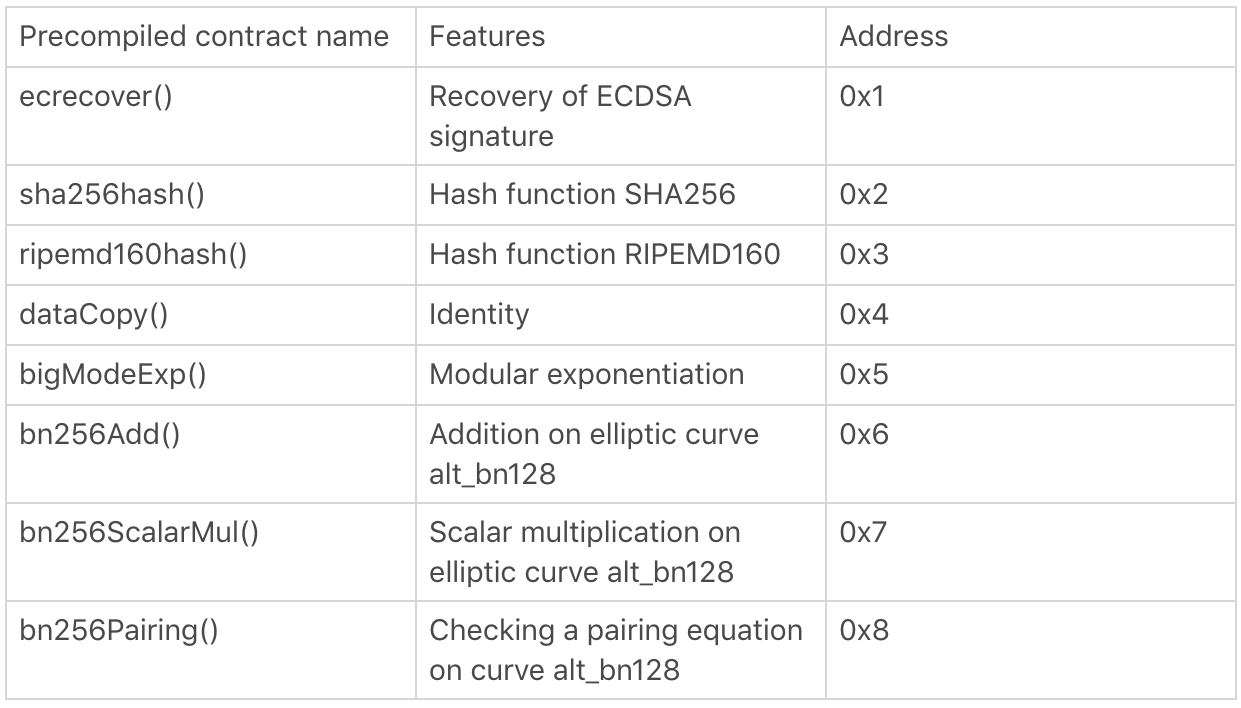# 以太坊预编译合约``````// run runs the given contract and takes care of running precompiles with a fallback to the byte code interpreter.
func run(evm *EVM, contract *Contract, input []byte, readOnly bool) ([]byte, error) {
if evm.ChainConfig().IsByzantium(evm.BlockNumber) {
precompiles = PrecompiledContractsByzantium
}
if p := precompiles[*contract.CodeAddr]; p != nil {
return RunPrecompiledContract(p, input, contract)
}
}
for _, interpreter := range evm.interpreters {
if interpreter.CanRun(contract.Code) {
if evm.interpreter != interpreter {
// Ensure that the interpreter pointer is set back
// to its current value upon return.
defer func(i Interpreter) {
evm.interpreter = i
}(evm.interpreter)
evm.interpreter = interpreter
}
}
}
return nil, ErrNoCompatibleInterpreter
}
``````

``````// RunPrecompiledContract runs and evaluates the output of a precompiled contract.
func RunPrecompiledContract(p PrecompiledContract, input []byte, contract *Contract) (ret []byte, err error) {
gas := p.RequiredGas(input)
if contract.UseGas(gas) {
return p.Run(input)
}
return nil, ErrOutOfGas
}
``````
``````// bn256Add implements a native elliptic curve point addition.
// RequiredGas returns the gas required to execute the pre-compiled contract.
func (c *bn256Add) RequiredGas(input []byte) uint64 {
}
func (c *bn256Add) Run(input []byte) ([]byte, error) {
x, err := newCurvePoint(getData(input, 0, 64))
if err != nil {
return nil, err
}
y, err := newCurvePoint(getData(input, 64, 64))
if err != nil {
return nil, err
}
res := new(bn256.G1)
return res.Marshal(), nil
``````

``````assembly {
if iszero(call(gasLimit, contractAddress, value, input, inputLength, output, outputLength)) {
revert(0, 0)
}
}
``````

• 发表于 2019-10-14 19:03
• 阅读 ( 4967 )
• 学分 ( 10 )
• 分类：以太坊